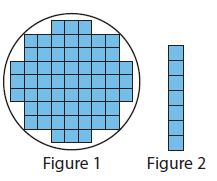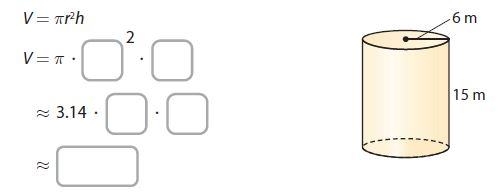Homework Explained - Math Practice 101Dear guest, you are not a registered member. As a guest, you only have read-only access to our books, tests and other practice materials.

As a registered member you can:

Registration is free and doesn't require any type of payment information. Click here to Register.
Go to page:
Chapter 13:Volume; Lesson 1: Volume of Cylinders

### Guided Practice

• Question 1

Vocabulary Describe the bases of a cylinder.

• Type below:
• Question 2

Figure 1 shows a view from above of inch cubes on the bottom of a cylinder. Figure 2 shows the highest stack of cubes that will fit inside the cylinder. Estimate the volume of the cylinder. Explain your reasoning.•  $$\text{ in}^3$$
• Question 3

Find the volume of the cylinder to the nearest tenth. Use 3.14 for $$\pi$$.The volume of the cylinder is approximately _____$$\text{ m }^3$$.

•  $$\text{ m}^3$$
• Question 4

A Japanese odaiko is a very large drum that is made by hollowing out a section of a tree trunk. A museum in Takayama City has three odaikos of similar size carved from a single tree trunk. The largest measures about 2.7 meters in both diameter and length, and weighs about 4.5 metric tons. Using the volume formula for a cylinder, approximate the volume of the drum to the nearest tenth.

The volume of the drum is about _____ $$\text{m} ^3$$.

•  The radius of the drum is about m The volume of the drum is about $$\text{ m}^3$$

### ESSENTIAL QUESTION CHECK-IN

• Question 5

How do you find the volume of a cylinder? Describe which measurements of a cylinder you need to know.

• Type below:

Yes, email page to my online tutor. (if you didn't add a tutor yet, you can add one here)